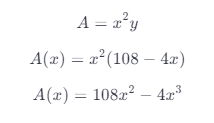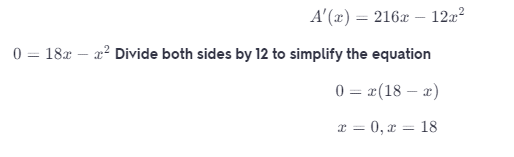# A Rectangular Package To Be Sent By A Postal Service Can Have A Maximum Combined Length And Girth

We thoroughly check each answer to a question to provide you with the most correct answers. Found a mistake? Let us know about it through the REPORT button at the bottom of the page.

A rectangular package to be sent by a postal service can have a maximum combined length and girth (perimeter of a cross-section) of 108 inches. Find the dimensions of the package of maximum volume that can be sent. (Assume the cross-section is square.)

Contents

108 – 4x*y

108-4x=yThere is a max at x = 18 because A'(x) = 0. There is a min at x = 0.

## Result

X=18

y(x)=108-4(18)=36